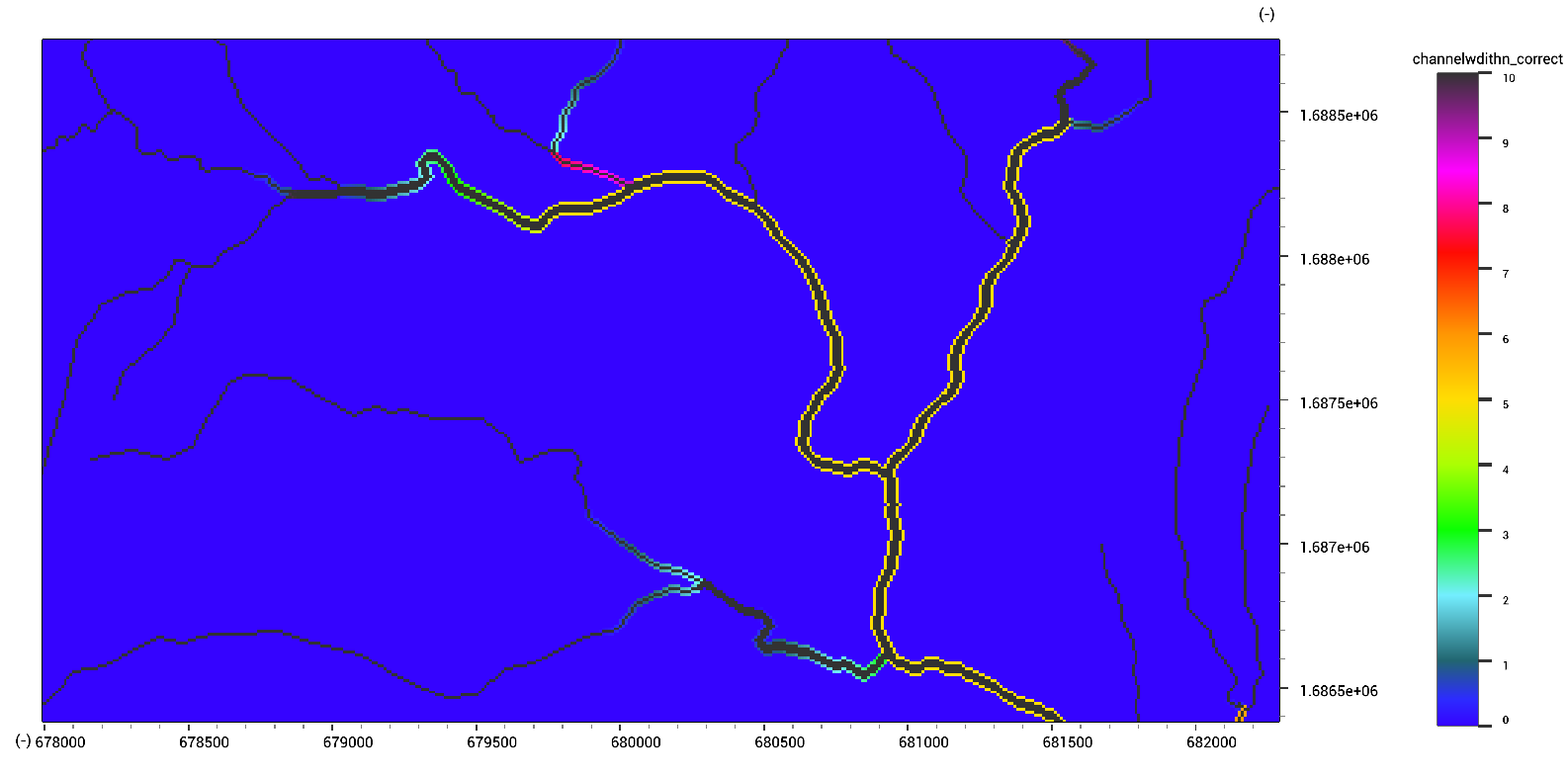LISEM Classic

LISEM

# Distances

The Spread (Approximate distance transform) function provides a rapid and flexible means of estimating distances (either actual or abstract). The function actually provides the lowest friction value from each cell to a source cell. Each source cell has a source friction value. All other cells have a travel friction cost.

channeldistance.map = Spread(channelmask.map,0.0,1.0)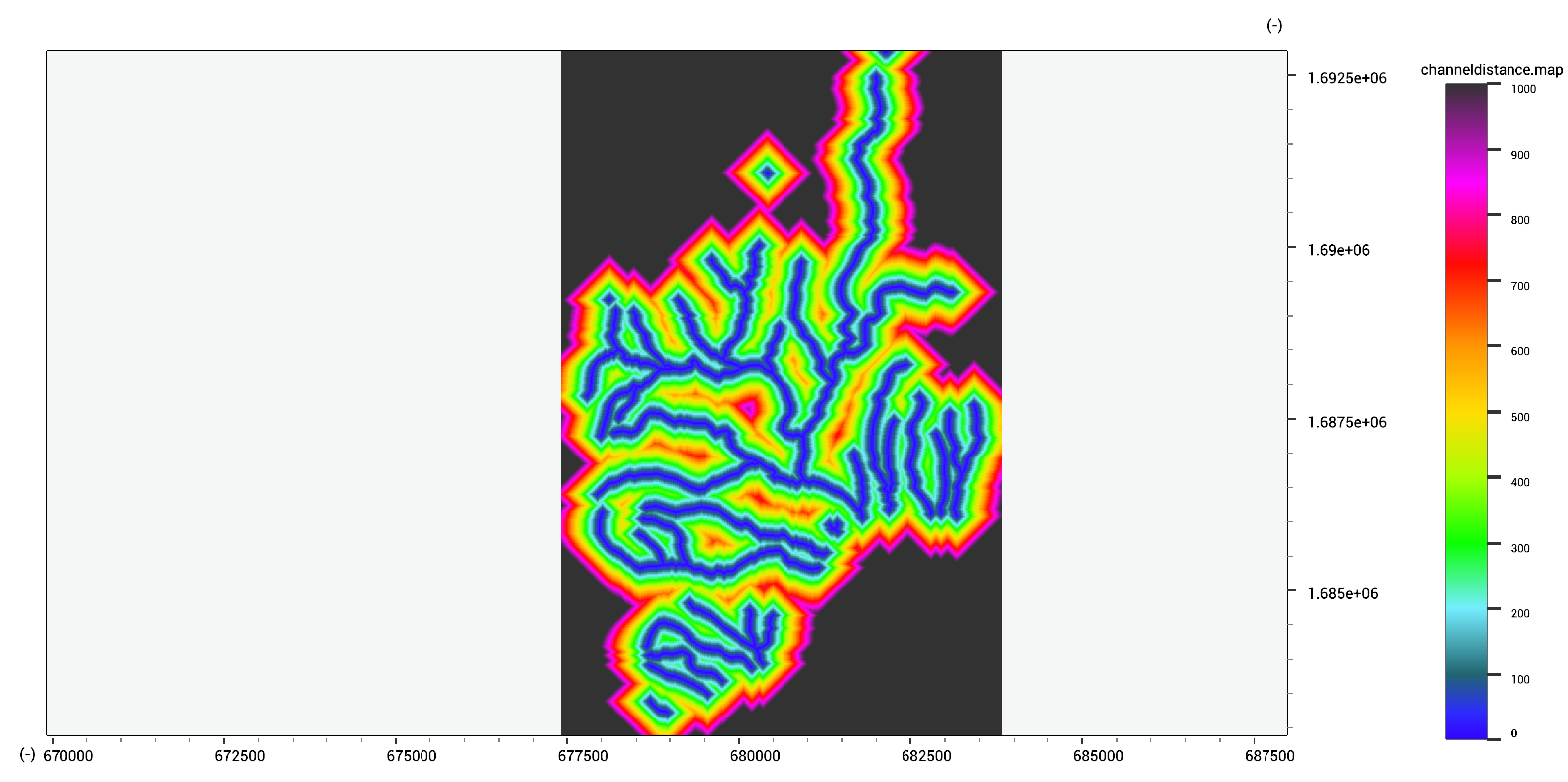A distance transform provides the actual euclidian distance to the closest source cell. Note that this function required inverted input (using the invert~ operator).

channeldistance.map = DistanceTransform(~channelmask.map)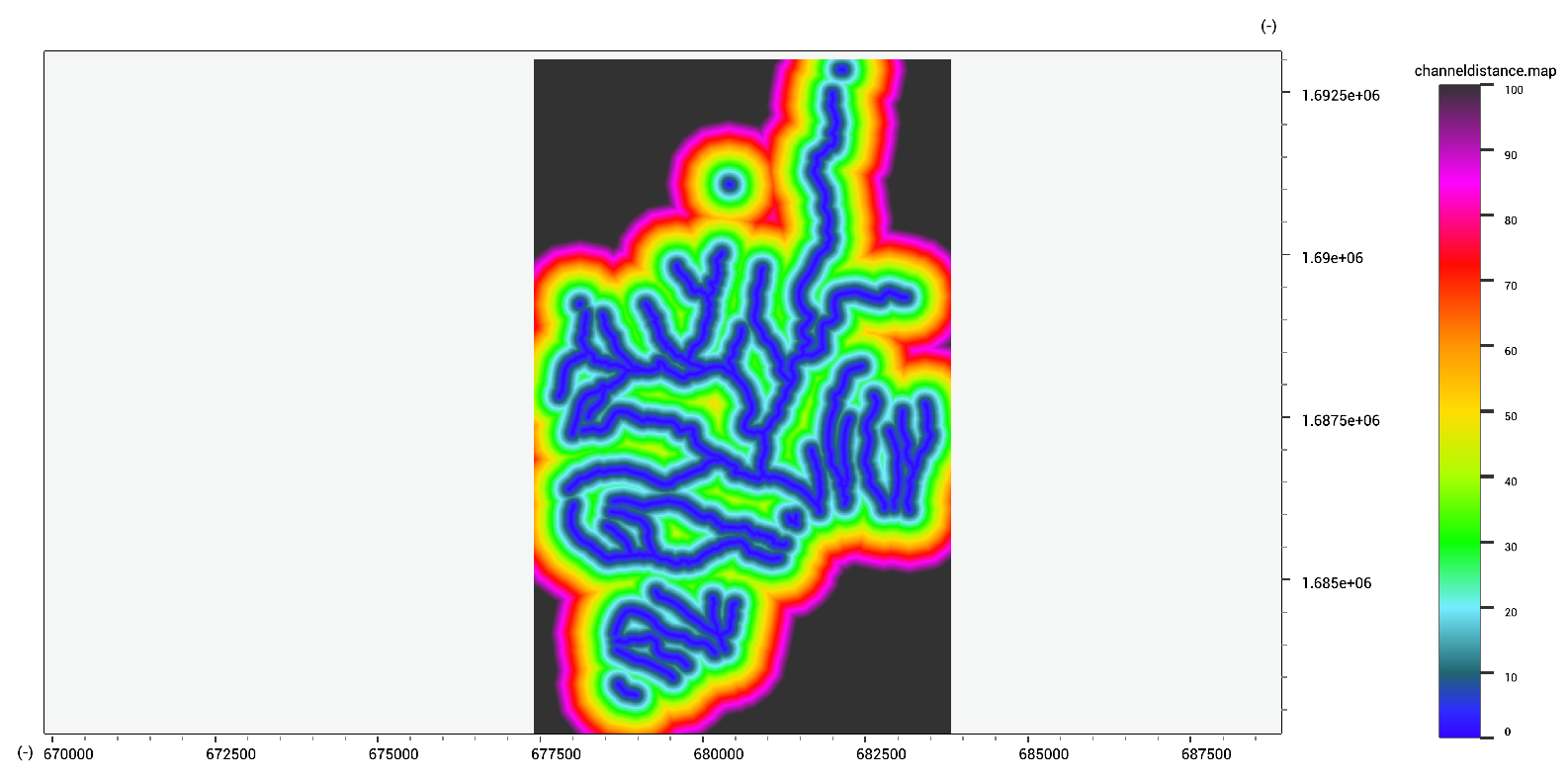Travel effort, or travel times, can be estimated by providing a spatially varying friction map. In this case, the friction on roads is low, and higher with steeper slopes. The friction on non-roads is significantly higher. The final travel effort map will show patterns of roads and terrain.

traveleffort.map = Spread(coast.map,0.0,MapIf(roadwidth.map >> 1,1 + slope.map,2 + 5 * slope.map))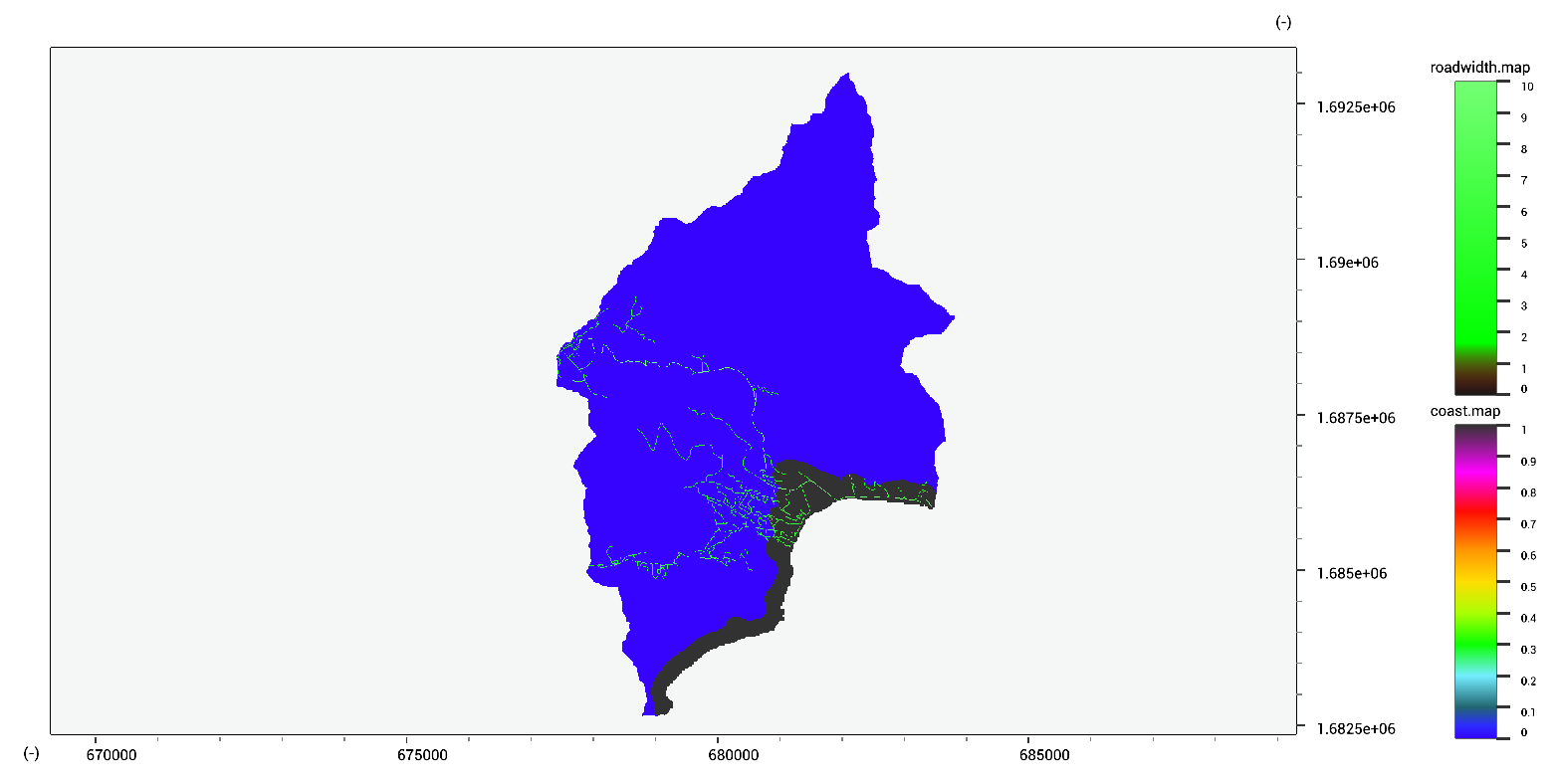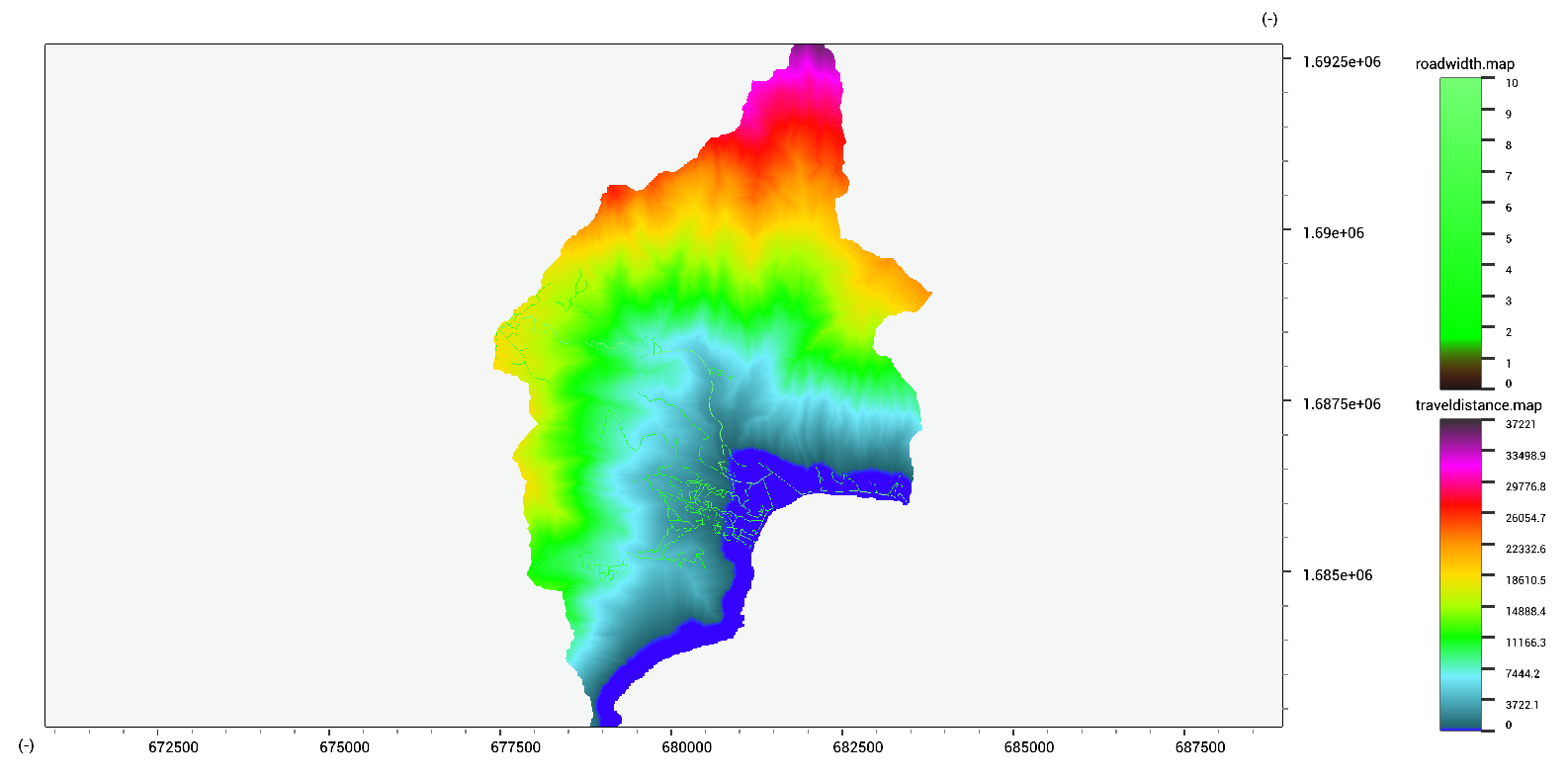When you have data on river width that exceeds the cellsize, you can distribute the channel over the neighboring cells using the spread function. There are some conversions going on due to the nature of the spread function. It starts with a certain source value, and spread to both sides equally.

channelwidth_new.map = max(0.0,min(-Spread(MapIf(channelwidth.map >> 0.0,1.0,0.0),-MapIf(channelwidth.map << 10.0, channelwidth.map,10.0+ (channelwidth.map-10)/2),1.0),10))